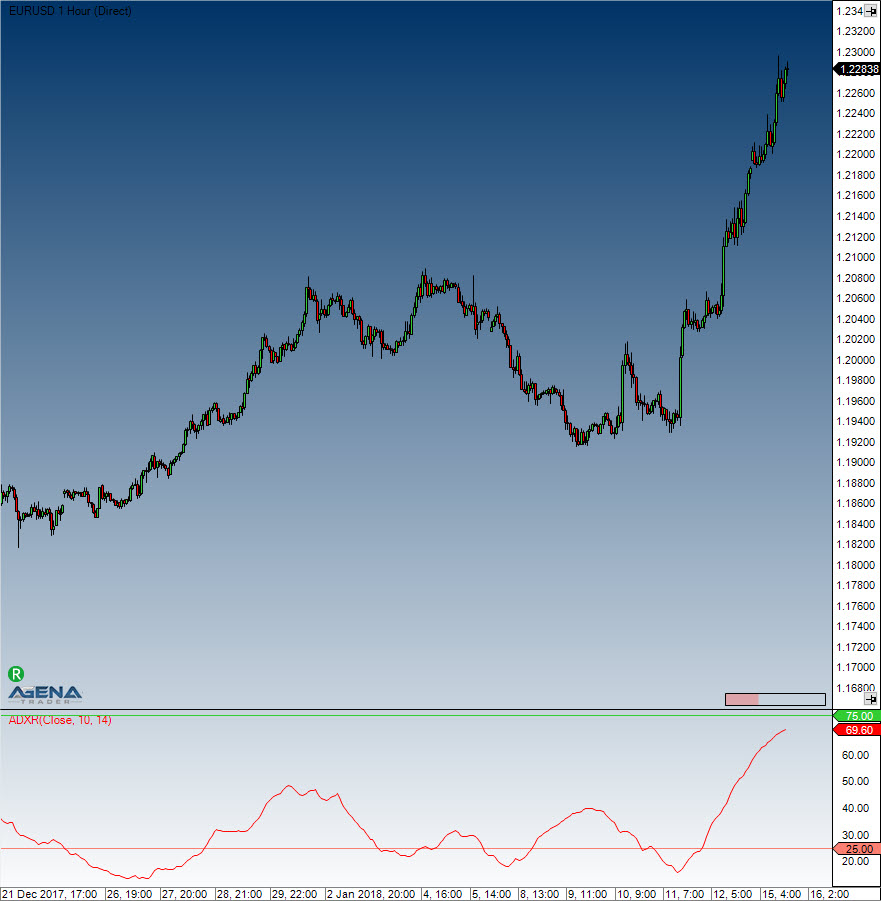# Average Directional Movement Rating (ADXR)

#### Description

The ADXR is the ADX indicator plus the ADX from n days ago divided by 2. Written as an equation, it looks like this: (current ADX + ADX n days ago) / 2.

#### Interpretation

The oscillator moves along a guiding line that typically has a value of 20. When the ADXR rises above 20, a trend exists. If the ADXR is below 20, no trend exists and the market is moving sideways. Welles Wilder recommends buying into the market at a value of 25 and higher, and holding the position as long as the value remains above 20.

#### Usage

ADXR(IDataSeries inSeries, int interval, int period)
ADXR(IDataSeries inSeries, int interval, int period)[int barsAgo]

#### Return value

double
When using this method with an index (e.g. ADXR(10, 14)[int barsAgo]), the value of the indicator will be outputted for the referenced bar.

#### Parameters

inSeries Input data series for the indicator
interval Interval between the first ADX value and the current ADX value
period Number of bars included in the calculation

#### Visualization